# 6.4 定制用户自己的邻居采样器¶

(English Version)

$\begin{split}\begin{gathered} \boldsymbol{a}_v^{(l)} = \rho^{(l)} \left( \left\lbrace \boldsymbol{h}_u^{(l-1)} : u \in \mathcal{N} \left( v \right) \right\rbrace \right) \\ \boldsymbol{h}_v^{(l)} = \phi^{(l)} \left( \boldsymbol{h}_v^{(l-1)}, \boldsymbol{a}_v^{(l)} \right) \end{gathered}\end{split}$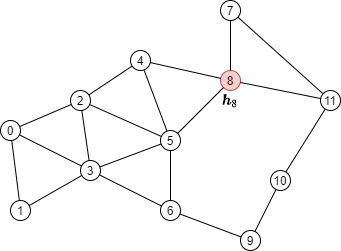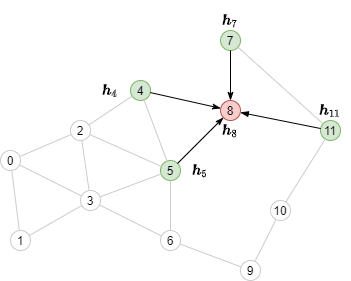## 理解邻居采样的工作原理¶

import torch
import dgl

src = torch.LongTensor(
[0, 0, 0, 1, 2, 2, 2, 3, 3, 4, 4, 5, 5, 6, 7, 7, 8, 9, 10,
1, 2, 3, 3, 3, 4, 5, 5, 6, 5, 8, 6, 8, 9, 8, 11, 11, 10, 11])
dst = torch.LongTensor(
[1, 2, 3, 3, 3, 4, 5, 5, 6, 5, 8, 6, 8, 9, 8, 11, 11, 10, 11,
0, 0, 0, 1, 2, 2, 2, 3, 3, 4, 4, 5, 5, 6, 7, 7, 8, 9, 10])
g = dgl.graph((src, dst))


### 找出消息传递的依赖¶$\begin{split}\begin{gathered} \boldsymbol{a}_8^{(2)} = \rho^{(2)} \left( \left\lbrace \boldsymbol{h}_u^{(1)} : u \in \mathcal{N} \left( 8 \right) \right\rbrace \right) = \rho^{(2)} \left( \left\lbrace \boldsymbol{h}_4^{(1)}, \boldsymbol{h}_5^{(1)}, \boldsymbol{h}_7^{(1)}, \boldsymbol{h}_{11}^{(1)} \right\rbrace \right) \\ \boldsymbol{h}_8^{(2)} = \phi^{(2)} \left( \boldsymbol{h}_8^{(1)}, \boldsymbol{a}_8^{(2)} \right) \end{gathered}\end{split}$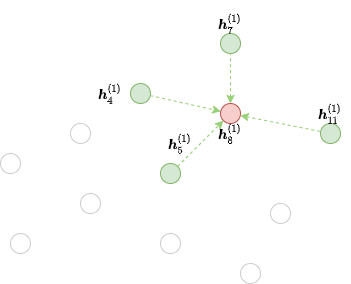DGL实现了多个可用于生成边界的函数。例如， dgl.in_subgraph() 是一个生成子图的函数，该子图包括初始图中的所有节点和指定节点的入边。 用户可以将其用作沿所有入边传递消息的边界。

frontier = dgl.in_subgraph(g, )
print(frontier.all_edges())


### 多层小批量消息传递的二分计算图¶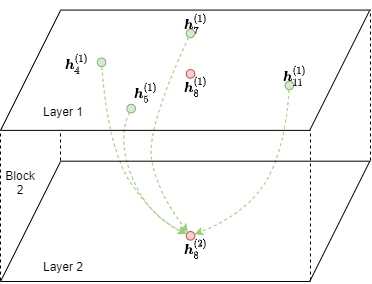DGL提供了 dgl.to_block() 以将任何边界转换为块。其中第一个参数指定边界， 第二个参数指定输出节点。例如，可以使用以下代码将上述边界转换为输出节点为8的块。

output_nodes = torch.LongTensor()
block = dgl.to_block(frontier, output_nodes)


num_input_nodes, num_output_nodes = block.number_of_src_nodes(), block.number_of_dst_nodes()
print(num_input_nodes, num_output_nodes)


block.srcdata['h'] = torch.randn(num_input_nodes, 5)
block.dstdata['h'] = torch.randn(num_output_nodes, 5)


print(block.srcdata['x'])
print(block.dstdata['y'])


DGL确保块的输出节点将始终出现在输入节点中。如下代码所演示的，在输入节点中，输出节点的ID位于其它节点之前。

input_nodes = block.srcdata[dgl.NID]
output_nodes = block.dstdata[dgl.NID]
assert torch.equal(input_nodes[:len(output_nodes)], output_nodes)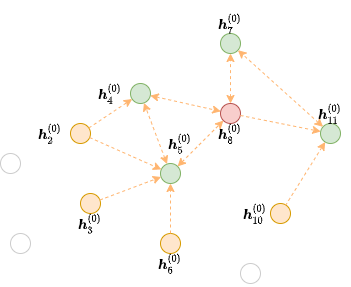dgl.to_block(frontier2, torch.LongTensor([4, 5]))   # ERROR


# 节点3是一个孤立节点，没有任何指向它的边.
block3 = dgl.to_block(frontier2, torch.LongTensor([4, 5, 7, 8, 11, 3]))
print(block3.srcdata[dgl.NID])
print(block3.dstdata[dgl.NID])


### 异构图上的采样¶

hetero_frontier = dgl.heterograph({
('user', 'follow', 'user'): ([1, 3, 7], [3, 6, 8]),
('user', 'play', 'game'): ([5, 5, 4], [6, 6, 2]),
('game', 'played-by', 'user'): (, )
}, num_nodes_dict={'user': 10, 'game': 10})


hetero_block = dgl.to_block(hetero_frontier, {'user': [3, 6, 8], 'block': [2, 6]})


# 输入的User和Game节点
print(hetero_block.srcnodes['user'].data[dgl.NID], hetero_block.srcnodes['game'].data[dgl.NID])
# 输出的User和Game节点
print(hetero_block.dstnodes['user'].data[dgl.NID], hetero_block.dstnodes['game'].data[dgl.NID])


## 实现一个自定义邻居采样器¶

sampler = dgl.dataloading.MultiLayerFullNeighborSampler(2)


BlockSampler 负责使用 sample_blocks() 方法从最后一层开始生成一个块的列表。 sample_blocks 的默认实现是向后迭代，生成边界，并将其转换为块。

class MultiLayerFullNeighborSampler(dgl.dataloading.BlockSampler):
def __init__(self, n_layers):
super().__init__(n_layers)

def sample_frontier(self, block_id, g, seed_nodes):
frontier = dgl.in_subgraph(g, seed_nodes)
return frontier


dgl.dataloading.neighbor.MultiLayerNeighborSampler 是一个更复杂的邻居采样器类，它允许用户为每个节点采样部分邻居节点以汇聚信息，如下所示。

class MultiLayerNeighborSampler(dgl.dataloading.BlockSampler):
def __init__(self, fanouts):
super().__init__(len(fanouts))

self.fanouts = fanouts

def sample_frontier(self, block_id, g, seed_nodes):
fanout = self.fanouts[block_id]
if fanout is None:
frontier = dgl.in_subgraph(g, seed_nodes)
else:
frontier = dgl.sampling.sample_neighbors(g, seed_nodes, fanout)
return frontier


class MultiLayerDropoutSampler(dgl.dataloading.BlockSampler):
def __init__(self, p, num_layers):
super().__init__(num_layers)

self.p = p

def sample_frontier(self, block_id, g, seed_nodes, *args, **kwargs):
# 获取种 seed_nodes 的所有入边
src, dst = dgl.in_subgraph(g, seed_nodes).all_edges()
# 以概率p随机选择边
# 返回一个与初始图有相同节点的边界
frontier = dgl.graph((src, dst), num_nodes=g.number_of_nodes())
return frontier

def __len__(self):
return self.num_layers


sampler = MultiLayerDropoutSampler(0.5, 2)
g, train_nids, sampler,
batch_size=1024,
shuffle=True,
drop_last=False,
num_workers=4)

model = StochasticTwoLayerRGCN(in_features, hidden_features, out_features)
model = model.cuda()

blocks = [b.to(torch.device('cuda')) for b in blocks]
input_features = blocks.srcdata     # 返回一个字典
output_labels = blocks[-1].dstdata     # 返回一个字典
output_predictions = model(blocks, input_features)
loss = compute_loss(output_labels, output_predictions)
loss.backward()
opt.step()


### 异构图上自定义采样器¶

class MultiLayerDropoutSampler(dgl.dataloading.BlockSampler):
def __init__(self, p, num_layers):
super().__init__(num_layers)

self.p = p

def sample_frontier(self, block_id, g, seed_nodes, *args, **kwargs):
# 获取 seed_nodes 的所有入边
sg = dgl.in_subgraph(g, seed_nodes)